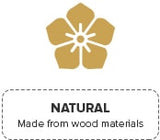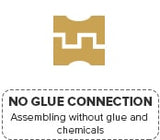# Safe - build your own working model by UGears

133
£46.99
Safety Comes First! If your favorite pastime is tinkering with construction kits and coming up with a neat creation, rest assured the Safe will more than satisfy your artistic urge....
Availability: 92 In Stock

Safety Comes First!

If your favorite pastime is tinkering with construction kits and coming up with a neat creation, rest assured the Safe will more than satisfy your artistic urge. This is a 3D puzzle safe that has a real combination lock on the front – it is an authentic replica of its real world counterpart. Wouldn’t these projects be a great way to occupy time on a rainy day or no TV night? On the other hand, if you wish to take up safe-cracking as a full-time professional, you can practice using this model.

• 100% hassle-free model – you don’t have to bother about putting in fresh batteries, gluing the parts together, or using any special tools.
• Made using premium grade plywood, this is an extraordinary model of a safe that can be used to store your money, documents, etc. properly.
• Still not convinced? Once you assemble the pieces, it becomes a real safe that opens with a traditional 3 figure coded lock. The unique combination is included in the kit. While closing the safe, you just have to shut the door and turn the handle in an anticlockwise direction.
• This construction kit is a fantastic gift for occasions like birthdays or holidays. You can turn into a friendly competition by letting others have a go at safe cracking, and awarding a prize to the one who is able to figure out the mechanism.

Be patient while assembling the safe – we assure you the end result is worth the effort and you can flaunt your skill as well. No longer do you need to worry about misplacing smaller items and trinkets – you can keep them locked away and safe. It will spare you the trouble of hunting in several places, because now you know exactly where your valuables are. Please hurry and order now!

How to create a custom lock combination for the model Safe?
Let’s suppose you would like to put the code 321:
A=3, B=2, C=1
(Technically number C - can be only 1, 2, 6 or 7)
P1, P2, P3 - plugs in the discs
Formula:
P3 - P2 = B - A + 8 (mod 10)
P1 = P2 + B – C (mod 10)

where A, B and C are the digits of the wanted code.

Safe’s code formula for 321 code:
P3 - P2 = 2 - 3 + 8
P1 = P2 + 2 - 1
P3 - P2 = 7
P1 = P2 + 1

Just choose one with P2 and P3 being 0 to 9

In this case you should use matching method to find the correct values. As you can see, this system of equations satisfy such values P1 = 1, P2 = 0, P3 = 7 (plugs in the discs)

Size models in finished form: 7.7*7.3*6.9 in (196 * 185 * 176 mm)

Inside space of  the safe : The  W*H*D   6.1*5.5*4.1 in  (155 * 140 * 105 mm)

Package size: 14.6*5.5*1.6 in  (37*14*4cm)

Number of parts: 179Answering the latest request on UGEARS Facebook page. Hope it will be interesting to you as well
***************************************************
What’s is the combination of plugs in the discs of Safe model for the wanted code 426?
***************************************************
Let’s suppose you would like to put the code 426:
A=4, B=2, C=6
The Formula for setting plugs in the discs:
a) P2 = P3 – ((B – A + 8) mod 10)
b) P1 = P2 + (B – C) mod 10
where A, B and C are the digits of the wanted code.
For technical reasons, C can only be 1, 2, 6 or 7
P1, P2, P3 – plugs in the discs. For technical reasons, P3 – P2 ≠ 4
E.g. let’s find P1, P2, P3 for the wanted code 426:
1.
Complete formula a) step by step:
P2 = P3 – ((B – A + 8) mod 10)
P2 = P3 – ((2 – 4 + 8) mod 10)
P2 = P3 – (6 mod 10)
P2 = P3 – 6
If you don’t now ‘mod 10’, than just go to Google an type ‘6 mod 10’ and Google will tell you that it’s 6
2.
Set in valid values, there are many possible solutions. Just choose one with P2 and P3 being 0 to 9
P2 = P3 – 6
with P3 = 6, P2 is 0
with P3 = 7, P2 is 1
with P3 = 8, P2 is 2
with P3 = 9, P2 is 3
3.
Solve formula b) with the first of the possible combinations
We will use P3: 6 and P2: 0
P1 = (P2 + B – C) mod 10
P1 = (0 + 2 – 6) mod 10
P1 = -4 mod 10
P1 = 6
again: Google will tell you, that “-4 mod 10” is 6, just don’t think about what this means
Solve formula b) with the second of the possible combinations:
We will use P3: 7 and P2: 1
P1 = (P2 + B – C) mod 10
P1 = (1 + 2 – 6) mod 10
P1 = -3 mod 10
P1 = 7
again: Google will tell you, that -3 mod 10 is 7, just don’t think about what this means
Solve formula b) with the third of the possible combinations:
We will use P3: 8 and P2: 2
P1 = (P2 + B – C) mod 10
P1 = (2 + 2 – 6) mod 10
P1 = -2 mod 10
P1 = 8
again: Google will tell you, that -2 mod 10 is 8, just don’t think about what this means
Solve formula b) with the last of the possible combinations:
We will use P3: 9 and P2: 3
P1 = (P2 + B – C) mod 10
P1 = (3 + 2 – 6) mod 10
P1 = -1 mod 10
P1 = 9
again: Google will tell you, that -1 mod 10 is 9, just don’t think about what this means
So: for 426, use 606 or 717 or 828 or 939 for plugs in the discs.
At last, make sure to set the parts together with the right way – as told in the manual. See is important when the wheels are put into the safe (For technical reasons, C can only be 1, 2, 6 or 7)